×#### Thank you for registering.

One of our academic counsellors will contact you within 1 working day.

Click to Chat

1800-1023-196

+91-120-4616500

CART 0

• 0

MY CART (5)

Use Coupon: CART20 and get 20% off on all online Study Material

ITEM
DETAILS
MRP
DISCOUNT
FINAL PRICE
Total Price: Rs.

There are no items in this cart.
Continue Shopping• Complete JEE Main/Advanced Course and Test Series
• OFFERED PRICE: Rs. 15,900
• View Details

```Chapter 11: Coordinate Geometry Exercise – 11.1

Question: 1

Plot the following points on the graph paper:

(i) (2, 5)

(ii) (4,- 3)

(iii) (- 5,- 7)

(iv) (7, - 4)

(v) (-3, 2)

(vi) (7, 0)

(vii) (- 4, 0)

(viii) (0, 7)

(ix) (0, - 4)

(x) (0, 0)

Solution:

The given points are,

A (2, 5), B (4, -3), C (-5, -7), D (7, - 4), E (- 3, 2),

F (7, 0), G (- 4, 0), H (0, 7), I (0, - 4), J (0, 0)

Let X 'OX and Y ' OY be the coordinate axes.

(i) Here for the given point the abscissa is 2 units and ordinate is 5 units. The point is in the first quadrant. So it will look like as shown in the following figure.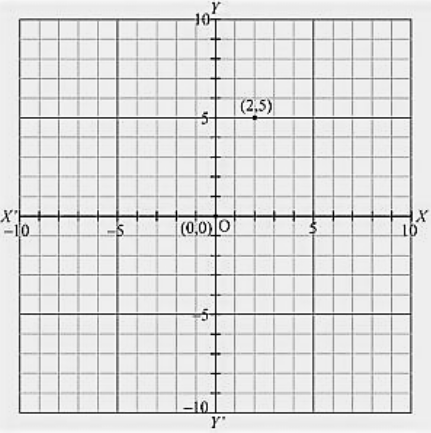(ii) Here for the given point the abscissa is 4 units and ordinate is -3 units. The point is in the fourth quadrant. So it will look like as shown in the following figure.(iii) Here for the given point the abscissa is -5 units and ordinate is -7 units. The point is in the third quadrant. So it will look like as shown in the following figure.(iv) Here for the given point the abscissa is 7 units and ordinate is -4 units. The point is in the fourth quadrant. So it will look like as shown in the following figure.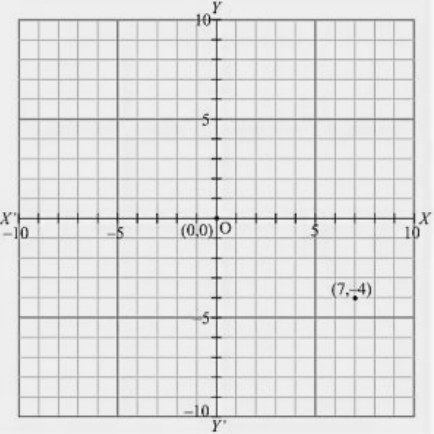(v) Here for the given point the abscissa is -3 units and ordinate is 2 units. The point is in the second quadrant. So it will look like as shown in the following figure.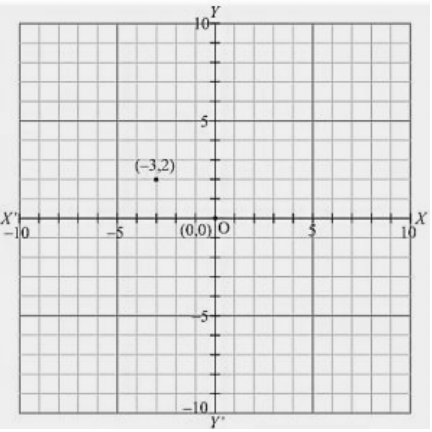(vi) Here for the given point the abscissa is 7 units and ordinate is 0 units. The point is on the x-axis. So it will look like as shown in the following figure.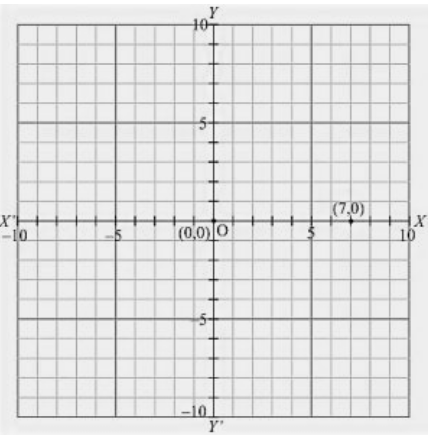(vii) Here for the given point the abscissa is - 4 units and ordinate is 0 units. The point is on the x-axis. So it will look like as shown in the following figure.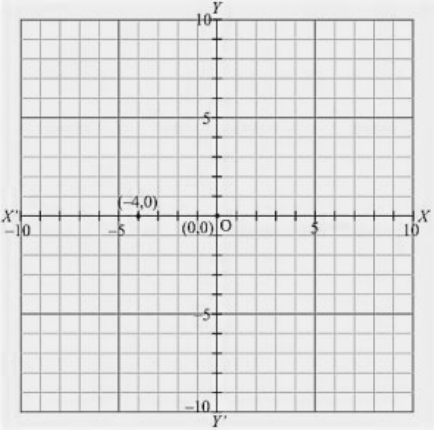(viii) Here for the given point the abscissa is 0 units and ordinate is 7 units. The point is on the y-axis. So it will look like as shown in the following figure.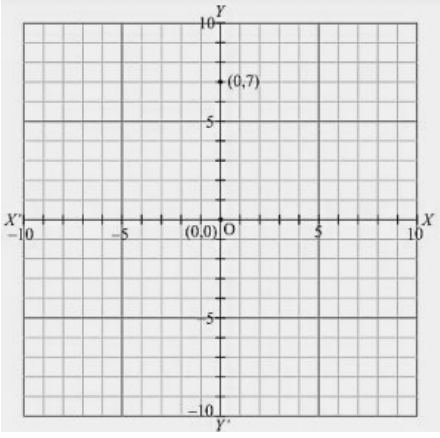(ix) Here for the given point the abscissa is 0 units and ordinate is -4 units. The point is on the y-axis. So it will look like as shown in the following figure.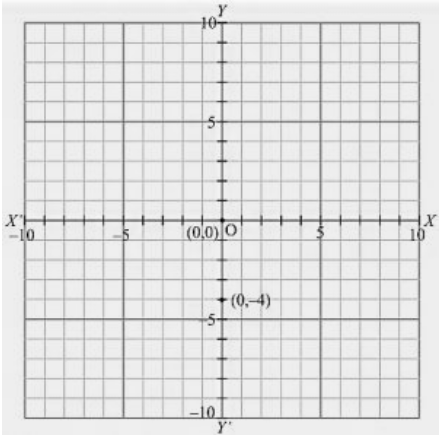(x) Here for the given point the abscissa is 0 units and ordinate is 0 units. The point is basically intersection of the coordinate axis. So it will look like as shown in the following figureQuestion: 2

Write the coordinates of each of the following points marked in the graph paper.Solution:

A (3, 1), B (6, 0), C (0, 6), D (-3, 0), E (- 4, 3), F (- 2, - 4), G (0, - 5), H (3,- 6), P (7, - 3), Q (7, 6)

1) The distance of point A from y-axis is 3 units and that of from x-axis is 1 units. Since A lies in the first quadrant, so its coordinates are (3, 1).

2) The distance of point B from y-axis is 6 units and that of from x-axis is 0 units. Since B lies on x-axis, so its coordinates are (6, 0).

3) The distance of point C from y-axis is 0 units and that of from x-axis is 6 units. Since C lies on y-axis, so its coordinates are (0, 6).

4) The distance of point D from y-axis is -3 units and that of from x-axis is 0 units. Since D lies on x-axis, so its coordinates are (-3, 0).

5) The distance of point E from y-axis is – 4 units and that of from x-axis is 3 units. Since E lies in the second quadrant, so its coordinates are (- 4, 3).

6) The distance of point F from y-axis is – 2 units and that of from x-axis is – 4 units. Since F lies in the third quadrant, so its coordinates are (- 2,- 4).

7) The distance of point G from y-axis is 0 units and that of from x-axis is – 5 units. Since G lies on y-axis, so its coordinates are (0,- 5).

8) The distance of point H from y-axis is 3 units and that of from x-axis is - 6 units. Since H lies in the fourth quadrant, so its coordinates are (3, - 6).

9) The distance of point P from y-axis is 7 units and that of from x-axis is - 3 units. Since P lies in the fourth quadrant, so its coordinates are (7,- 3).

10) The distance of point Q from y-axis is 7 units and that of from x-axis is 6 units. Since Q lies in the first quadrant, so its coordinates are (7, 6).
```### Course Features

• 728 Video Lectures
• Revision Notes
• Previous Year Papers
• Mind Map
• Study Planner
• NCERT Solutions
• Discussion Forum
• Test paper with Video Solution